# RD Sharma Class 10 Ex 3.10 Solutions Chapter 3 Pair of Linear Equations in Two Variables

In this chapter, we provide RD Sharma Class 10 Ex 3.10 Solutions Chapter 3 Pair of Linear Equations in Two Variables for English medium students, Which will very helpful for every student in their exams. Students can download the latest RD Sharma Class 10 Ex 3.10 Solutions Chapter 3 Pair of Linear Equations in Two Variables pdf, Now you will get step by step solution to each question.

# Chapter 3: Pair of Linear Equations in Two Variables Exercise – 3.10

### Question: 1

Points A and B are 70 km. apart on a highway. A car starts from A and another car starts from B simultaneously. If they travel in the same direction, they meet in 7hrs, but if they travel towards each other, they meet in one hour. Find the speed of two cars.

### Solution:

We have to find the speed of car

Let x and y be two cars starting from points A and B respectively. Let the speed of car x be x km/hr and that of car y be y km/hr.

Case I:

When two cars move in the same directions:

Suppose two cars meet at point Q, Then,

Distance travelled by car X = AQ

Distance travelled by car Y = BQ

It is given that two cars meet in 7 hours.

Therefore, Distance travelled by car X in 7 hours = 7x km

AQ = 7x

Distance traveled by car y in 7 hours = 7y km

BQ = 7Y

Clearly AQ – BQ = AB

7x – 7 y = 70 Dividing both sides by common factor 7 we get,

x – y = 10 .  …… (I)

Case II :

When two cars move in opposite direction

Suppose two cars meet at point. Then,

Distance travelled by car x = AP,

Distance travelled by car y = BP,

In this case, two cars meet in 1 hour

Therefore Distance travelled by car X in 1 hour =1x km

AP = Ix

Distance travelled by car Y in 1 hour = ly km

BP = ly

AP + BP = AB

AP + BP = AB

x + y = 70 … (ii)

By solving equation (i) and (ii),

x = 40

Substituting x = 40 in equation (ii) we get

x + y = 70

40 + y = 70

y = 70 – 40

y = 30

Hence, the speed of car starting from point A is 40 km/hr.

The speed of car starting from point B is 30 km/hr.

### Question: 2

A sailor goes 8 km downstream in 40 minutes and returns in 1 hour. Determine the speed of the sailor in still water and the speed of the current.

### Solution:

Let the speed of the sailor in still water be x km/hr and the speed of the current be y km/hr

Speed upstream = (x- y) Km/hr

Speed downstream = (x + y) km/hr

Now, Time taken to cover 8 km downstream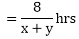Time taken to cover 8 km upstream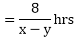But, time taken to cover 8 km downstream in 40 minutes or 40/60 hours that is 2/3 hrs.

8 × 3 = 2(x + y)

24 = 2x + 2y

Dividing both sides by common factor 2 we get

12 = x + y …… (1)

Time taken to cover 8km upstream in 1hour,

8 = 1(x – y)   …….. (2)

By solving these equation (i) and (ii) we get,

x = 10

Substitute x = 10 in equation (i) we get,

x + y = 12

10 + y = 12

y = 12 – 10

y = 2

Hence, the speed of sailor is 10 km/hr.

Speed of Current is 2 km/hr.

### Question: 3

The boat goes 30 km upstream and 44 km downstream in 10 hrs. In 13 hours it can go 40 km upstream and 55 km downstream. Determine the speed of stream and that of boat in still water.

### Solution:

Let the speed of the boat in still water be x km/hr and the speed of the stream be y km/hr

Speed upstream = (x – y) km/hr

Speed downstream = (x + y) km/hr Now,

Time taken to cover 30 km upstreamTime taken to cover 44 km downstream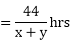But total time of journey is 10 hours

Time taken to cover 40 km upstream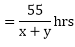In this case total time of journey is given to be 13 hours

Therefore,

Puttingin equation (i) and (ii) we get

30u + 44v = 10

40u + 55v = 10

30u + 44v – 10 = 0 …. (iii)

40u + 551 – 13 = 0 … (iv)

Solving these equations by cross multiplication we get,

Now,

1 × 10 = 2(x – y)

10 = 2x – 2y/2

v = 1/11

Substituting x = 8 in equation we get,

x + y = 11

8 + y = 11

y = 11 – 8

y = 3

Hence, speed of boat in still water is 8 km/hr.

Speed of the stream is 3 km/hr.

### Question: 4

A boat goes 24 km upstream and 28 km downstream in 6 hrs. It goes 30 km upstream and 21 km downstream in 6.5 hours. Find the speed of the boat in still water and also speed of the stream.

### Solution:

We have to find the speed of the boat in still water and speed of the stream

Let the speed of the boat in still water be x km/hr and the speed of the stream be y km/hr the

Speed upstream = (x – y) km/hr

Sped down stream= (x + y) km/hr

Now, Time taken to cover 28 km downstream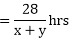Time taken to cover 24 km upstream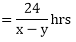But, total time of journey is 6 hours,

Time taken to cover 30 km upstream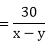Time taken to cover 21km downstream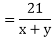In this case total time of journey is given to 6.5 hrs.

24u + 28v  – 6 = 0  … (iii)

30u + 21v – 13/2 = 0  … (iv)

Solving these equations by cross multiplication we get,

Now,

x + y = 14 … (eq)

By solving he equations,

x = 10

Substituting x = 10 in equation, we get,

Speed of the stream = 4 km/hr.

Speed of boat = 10 km/hr.

### Question: 5

A man walks a certain distance with certain speed. If he walks ½ km an hour faster, he takes 1 hour less. But, if he walks 1km an hour slower, he takes 3 more hours. Find the distance

### Solution:

Let r = the rate the man walked

then (r + 0.5) = 1 hr less rate

and (r – 1) = 3 hr more rate

let t = time he actually walked,

then (t – 1) = time taken at the faster speed

and (t + 3) = time at the slower speed

Distance for all three situations is the same (dist = rate x time):

So, rt = (r + 0.5)(t – 1)

rt = rt – r + 05t – 0.5

rt – rt + r – 0.5t = -0.5

r – 0.5t = -0.5 (1)

Also, for the slower speed case,

rt = (r – 1)(t + 3)

rt = rt + 3r – t – 3

rt – rt – 3r + t = -3

-3r + t = -3(2)

Multiply the 1st equation by 2 and add to the above equation

2r – t = – 1

-3r + t = – 3

– r = – 4

r = 4 km/h is the actual walking rate

Find t using: r – 0.5t = – 0.5

4 – 0.5t = – 0.5

– 0.5t = -0.5 – 4

– 0.5t = – 4.5

t = 9 hrs actual time

Hence, Distance = r × t

4 × 9 = 36 km.

### Question: 6

A person rowing at the rate of 5 km/hr in still water takes thrice as much as time in going 40 km upstream as in going 40 km downstream. Find the speed of the stream.

### Solution:

Let x = the rate water is flowing

Then,

(5 + x) = boat speed downstream

and

(5 – x) = boat speed upstream

Let d = distance one way

write a time equation;

Upstream time = 3 times downstream time

Cross multiply

d(5 + x) = 3d(5 – x)

divide both sides d and eliminate it:

5 + x = 3(5 – x)

5 + x = 15 – 3x

x + 3x = 15 – 5

4x = 10

x = 10/4

x = 2.5 km/hr is the rate of the current.

### Question: 7

Ramesh travels 760 km to his home partly by train and partly by car. He takes 8hours if he travels 160 km, by train and the rest by car. He takes 12 minutes more if he travels 240 km by train and the rest by car. Find the speed of train and the car respectively.

### Solution:

Let the speed of the train be x km/hour that of the car be y km/hr, we have the following cases

Case I:

When Ramesh travels 760 Km by train and the rest by car

Time taken by Ramesh to travel 160 Km by train = 160/x hrs

Total time taken by Ramesh to cover 760 Km

It is given that total time taken in 8 hours

Time taken by Ramesh to travel

It is given that total time taken in 8 hours

Case II:

When Ramesh travels 240 Km by train and the rest by car

Time taken by Ramesh to travel 240 Km by train = 240/x hrs

Time taken by Ramesh to travel (760 – 240) = 520 Km by car = 520/y hrs

In this case total time of the journey is 8 hours 12 minutes

Now,

x = 80.

And

v = 1/100

1/y = 1/100

y = 100

Hence, the speed of the train is 80 km/hr.

Speed of car = 100 km/hr.

### Question: 8

A man travels 600 km partly by train and partly by car. If he covers 400 km by train and the rest by car, it takes him 6 hours and 30 minutes. But, if he travels 200 km by train and the rest by car, he takes half an hour longer. Find the speed of the train and the speed of the car.

### Solution:

Let the speed of the train be x km/hr that of the car be y km/hr, we have following cases,

Case 1:

When a man travels 600km by train and the rest by car,

Time taken by a man to travel 400 km by train = 400/x hrs

Time taken by a man to cover 600 km

It is given that the total time taken in 8 hours,

Case II

When a man travels 200 km by train and rest by car

TT by man to travel 200 km = 200/x hrs

TT by man to travel 400 km = 400/y hrs

In this case, total time of the journey in 6 hours 30 minutes + 30 minutes that is 7 hrs,

Putting 1/x = u, and, 1/y = u,

Then,

By equating,

u = 1/100

By substituting u = 1/100 in the equation,

v = 180

Now,

x = 100

y = 80

Hence, the speed of the train is 100 km/hr.

The speed of the car is 80 km/hr.

### Question: 9

Places A and B are 80 km apart from each other on a highway. A car starts from A and other from B at the same time. If they move in the same direction, they meet in 8hours and if they move in opposite direction, they meet in 1hour and 20 minutes. Find the speeds of the cars.

### Solution:

Let x and y be two cars starting from points A and B respectively.

Let the speed of the car X be x km/hr and that of the car Y be y km/hr.

Case I:

When two cars move in the same directions:

Suppose two cars meet at point Q, then,

Distance travelled by car X = AQ

Distance travelled by car Y = BQ

It is given that two cars meet in 8 hours.

Distance travelled by car X in 8 hours =8x km.

AQ = 8x

Distance travelled by car Yin 8 hours =8y km

BQ = 8y

Clearly AQ – BQ = AB

8x – 8y = 80

Both sides divided by 8, we get

x – y = 10 .–(i)

Case II:

When two cars move in opposite direction

Suppose two cars meet at point P, then,

Distance travelled by X car X = AP

Distance travelled by Y car Y = BP

In this case, two cars meet in 1 hour 20 minutes, we can write it as 1 hour = 20/60 hours.

Therefore,

Distance travelled by a car Y in

x + y = 60 … (ii)

By asolving the equations, we get,

x = 35.

By substituting x = 35 in equation (ii), we get

x + y = 60

35 + y = 60

y = 60 – 35

y = 25.

Hence, speed of car x = 35 km/hr.

Speed of car y = 25 km/hr.

### Question: 10

A boat goes 12 km upstream and 40 km downstream in 8 hours. It can go 16 km upstream and 32 km downstream in the same time. Find the speed of the boat in still water and the speed of the stream.

### Solution:

We have to find the speed of the boat in still water and speed of the stream

Let the speed of the boat in still water be 1 km/hr and the speed of the stream be r km/hr then

Speed upstream = (x – y) km/hr

Sped down stream= (x + y) km/hr

Now, Time taken to cover 12 km upstream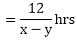Time taken to cover 40 km downstream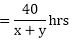But, total time of journey is 8 hours

Time taken to cover 16 km upstream

x – y Time taken to cover 32 km downstream

In this case total time of journey is given to 8 hrs

12u + 40 v = 8

16u + 32 v = 8

12u + 40v – 8 = 0 … (iii)

16u + 32v – 8 = 0 … (iv)

Solving these equations by cross multiplication, we get,

Now,

4 = x – y  …  (v)

And

x + y = 8  …. (vi)

By solving equation (v) and (vi) we get,

x = 6

x + y = 8

6 + y = 8

y = 8 – 6

y = 2

Hence, the speed of boat in still water is 6 km/hr.

The speed of the stream is 2 km/hr.

### Question: 11

Roohi travels 300 km to her home partly by train and partly by bus. She takes 4 hours if she travels 60 km by train and the remaining by bus. If she travels 100 kms by train and the remaining by bus, she takes 10 minutes longer. Find the speed of the train and the bus separately.

### Solution:

Let the speed of the train be x km/hr that of the bus be y km/hr, we have the following cases,

When Roohi travels 300km by train and rest by bus,

Time taken by Roohi to travel 60km by train = 60/x hrs

Time taken by Roohi to trave (300 – 60) = 240 km by bus = 240/y hrs

Total time taken by Rohi to cover 300 km

It is given that total time taken in 4 hours

Case II

When Roohi travels 100km by train and the rest by bus,

Time taken by Roohi to travel 100km by train = 100/x

Time taken by Roohi to travel (300 – 100) = 200 km by bus = 200/y

In this case total time of the journey is 4 hours 10 minutes

Subtracting equation (iv) from (iii) we get,

Now,

x = 60

And,

Hence, the speed of the train is 60 km/hr.

The speed of the bus is 80 km/hr.

### Question: 12

Ritu can row downstream 20 km in 2 hours, and upstream 4 km in 2 hours. Find her speed of rowing in still water and the spees of the current.

### Solution:

Let the speed of rowing be ‘X’ km/hr and the speed of the current be ‘Y’ km/hr,

Speed upstream = (x – y) km/hr.

Speed downstream = (x + y) km/hr.

Now,

Time taken to cover 20 km downstreamTime taken to cover 4 km upstream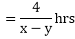But, time taken to cover 20 km downstream in 2 hours,

20 = 2(x + y)

20 = 2x + 2y … (i)

Time Taken to cover 20 km downstream in 2 hours

20 = 2(x + y)

20 = 2x + 2y … (ii)

By solving (i) and (ii) we get,

2y = 8

y = 4

Hence, speed of rowing in still water is 6 km/hr.

Speed of current is 4 km/hr.

### Question: 13

A mother boat can travel 30 km upstream and 28 km downstream in 7 hours. It can travel 21 km upstream and return in 5 hours. Find the speed of the boat in still water and speed of the stream.

### Solution:

Let the speed of A and B be X km/hr and Y km/hr respectively. Then,

Time taken by A to cover 30km = 30/x hrs,

And, time taken by B to cover 30km = 30/y hrs.

By the given conditions, we have,

If A doubles his pace, then speed of A is 2x km/hr

Time taken by A to cover 30 km = 30/2x hrs

Time taken by B to cover 30 km = 30/y hrs

According to given condition we have,

10u – 10v = 1

10u – 10v = 0 … (iii)

-10u + 20v = 1

-10u + 20v – 1 = 0 … (iv)

Adding equations (iii) and (iv), we get,

v = 1/5

Putting v = 1/5 in equation (iii), we get,

10u – 10v – 1 = 0

10u – 3 = 0

y = 5

Hence, the A’s speed is 10/3 km/hr.

Then B’s speed is 5 km/hr.

### Question: 14

Abdul travelled 300 km by train and 200 km by taxi, it took him 5 hours and 30 mins. But if he travels 260 km by train and 240 km by taxi he takes 6 mins longer. Find the speed of the train and that of the taxi.

### Solution:

Let the speed of the train be x km/hour that of the taxi be y km/hr, we have the following cases

Case I: When Abdul travels 300 Km by train and the 200 Km by taxi

Time taken by Abdul to travel 300 Km by train = 300/x hrs

Time taken by Abdul to travel 200 Km by taxi = 200/y

Total time taken by Abdul to cover 500 Km

It is given that total time taken in 5 hours 30 minutes

Case II:

When Abdul travels 260 km by train and the 240 km by taxi

Time taken by abdul to travel 260 km by train = 260/x hrs

Time taken by Abdul to travel 240 km by taxi = 240/y hrs

In this case, total time of the journey is 5 hours 36 minutes.

Putting 1/x = u and 1/y = v, the equations (i) and (ii) reduces to

Multiplying equation (iii) by 6, the above equation becomes

Subtracting equation (iv) from (v) we get

Putting u = 1/100 in equation (iii), we get

v = 1/2

v = 1/80

Now,

u = 1/100

x = 100

And,

y = 80

Hence, the speed of the train is 100 km/hr

The speed of the taxi is 80 km/hr.

### Question: 15

A train covered a certain distance at a uniform speed. If the train could have been 10 km/hr faster, it would have taken 2 hours less than the scheduled time and if the train were slower by 10 km/hr; it would have taken 3 hours more than the scheduled time. Find the distance covered by the train.

### Solution:

Let the actual speed of the train be x km/hr and the actual time is taken by y hours. Then,

Distance = Speed x Time

Distance covered = (xy) km … (i)

If the speed is increased by 10 Km I hr, then the time of journey is reduced by 2 hours.

When speed is (x + 10) km I hr, time of journey is (y – 2) hours.

Therefore, Distance covered = (x + 10) (y – 2)

xy = (x + 10)(y – 2)

xy = xy + 10y – 2x – 20

– 2x + 10y – 20 = 0

– 2x + 3y – 12 = 0 … (ii)

When the speed is reduced by 10 Km/hr, then the time of journey is increased by 3 hours.

When speed is (x -10) Km/h, time of journey is (y + 3) hours.

Hence, Distance covered = (x – 10) (y + 3)

xy = (x – 10)(y + 3)

0 = – 10y + 3x – 30

3x – 10y – 30 = 0 … (iii)

Thus, we obtain the following system of equations: = x + 5y – 10 = 0

x + 5y – 10 = 0

3x – 10y – 30 = 0

By using cross-multiplication, we have

y = 12

Putting the values of x and y in equation (i), we obtain

Distance = xy km

= 50 × 12

= 600 km

Hence, the length of the journey is 600 km

### Question: 16

Places A and B are 100 km apart on a highway. One car starts from A and another from B at the same time. If the cars travel in the same direction at different speeds they meet in 5 hours. If they travel towards each other, they meet in 1 hour. What are the speeds of two cars?

### Solution:

Let x and y be two cars starting from points A and B respectively.

Let the speed of the car X be x km/hr and that of the car Y be y km/hr.

Case I: When two cars move in the same directions: Suppose two cars meet at point Q, then,

Distance travelled by car X = AQ

Distance travelled by car Y = BQ

It is given that two cars meet in 5 hours.

Distance travelled by car X in 5 hours = 5x km AQ = 5x

Distance travelled by car Y in 5 hours = 5y km BQ = 5y

Clearly AQ – BQ = AB 5x – Sy = 100

Both sides divided by 5, we get x – y = 20 … (i)

Case II: When two cars move in opposite direction

Suppose two cars meet at point P, then,

Distance travelled by X car X = AP

Distance travelled by Y car Y = BP

In this case, two cars meet in 1 hour

Therefore, Distance traveled by car y in hours = lx km

Distance traveled by car y in 1 hours = ly km

AP + BP = AB

1x + 1y = 100

X + y = 100

By solving, x = 60,

Substituting x = 60 in equation, we get,

x + y = 100

60 + y = 100

Y = 40

Hence, the speed of car X is 60 km/hr.

Speed of car Y = 40 km/hr.

All Chapter RD Sharma Solutions For Class10 Maths

I think you got complete solutions for this chapter. If You have any queries regarding this chapter, please comment on the below section our subject teacher will answer you. We tried our best to give complete solutions so you got good marks in your exam.